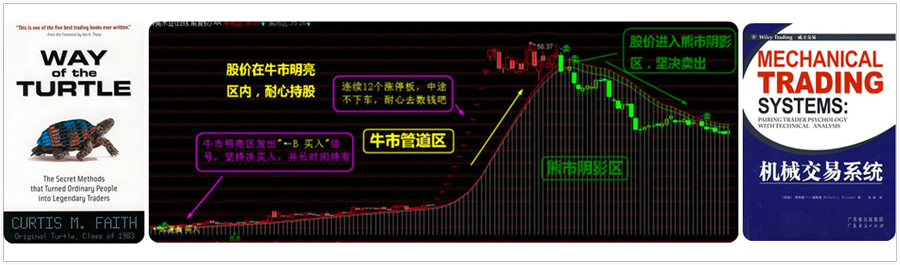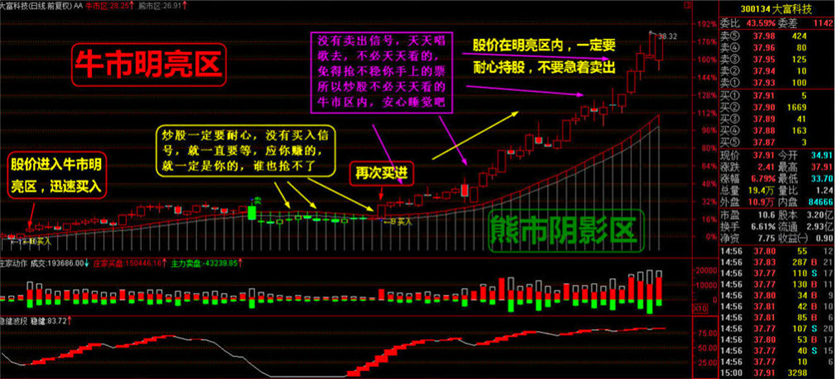# 通达信MACD二次金叉副图指标公式源码

2019-01-31 22:32:44  阅读 1095 次 评论 0 条DIFF:=EMA(CLOSE,12) - EMA(CLOSE,26);&nbsp; {选股设JC=10即可}
DEA:=EMA(DIFF,9);
MACD:=2*(DIFF-DEA),COLORSTICK;
A:=IF(CROSS(0,DEA),1,0);
A1:=IF(DIFF<0 AND CROSS(DIFF,DEA),1,0);
SS:=IF(A,SUM(A1,0),0);
SSB:=HHV(SS,0);
SSS:=SUM(A1,0);
JC:IF(SSS-SSB=2 AND SSS>REF(SSS,1),10,0);

 势至简高智稳系统免费下载： 本地免费下载